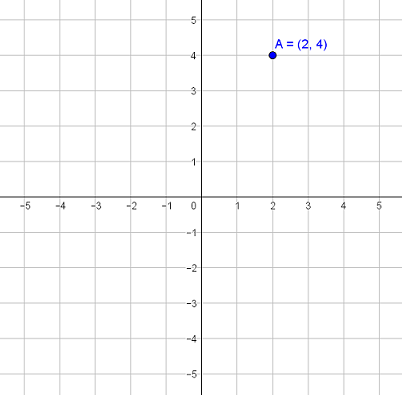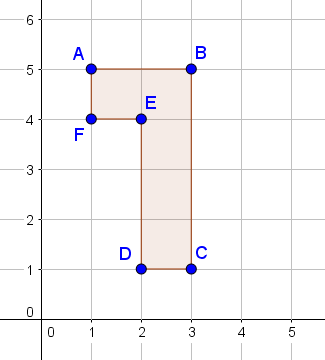Match Fishtank is now Fishtank Learning!

# Transformations and Angle Relationships

## Objective

Describe multiple rigid transformations using coordinate points.

## Common Core Standards

### Core Standards

?

• 8.G.A.2 — Understand that a two-dimensional figure is congruent to another if the second can be obtained from the first by a sequence of rotations, reflections, and translations; given two congruent figures, describe a sequence that exhibits the congruence between them.

• 8.G.A.3 — Describe the effect of dilations, translations, rotations, and reflections on two-dimensional figures using coordinates.

## Criteria for Success

?

1. Understand that a translation in the horizontal direction adds or subtracts to the $x-$coordinate, and a translation in the vertical direction adds or subtracts to the $y-$coordinate. In general, ${(x, y) \rightarrow (x+a, y+b)}$.
2. Understand that a reflection over the $x-$axis will keep the $x-$coordinate the same but have the opposite value of the $y-$coordinate, or ${(x,y) \rightarrow (x,-y)}$; and a reflection over the $y-$axis will keep the $y-$coordinate the same but have the opposite value of the $x-$coordinate, or ${(x,y) \rightarrow (-x,y)}$.
3. Determine new coordinates of points that undergo transformations.

## Tips for Teachers

?

• Students started some work with coordinate points and transformations in Lessons 3 and 5. This lesson extends on that to look at all three rigid transformations separately and in combination. Standard 8.G.3 will come back later in dilations.
• Students do not need to memorize the rules or the formal notation. The focus should be on understanding what impact a transformation has on the points on a figure and how that is represented through the values of the coordinates (MP.7). This will enable students to conceptualize these motions without using a coordinate plane.
• Use the coordinate plane as a support, with graph paper on hand, but encourage students to try problems first without it.

#### Remote Learning Guidance

If you need to adapt or shorten this lesson for remote learning, we suggest prioritizing Anchor Problem 1 (benefits from worked example). Find more guidance on adapting our math curriculum for remote learning here.

#### Fishtank Plus

• Problem Set
• Student Handout Editor
• Vocabulary Package

## Anchor Problems

?

### Problem 1

Point $A$ is located at ${ (2, 4)}$. Perform the following transformations on point $A$, and label each new point.

• Translate point $A$  $2$ units to the right and $4$ units down. Label it point $W$.
• Rotate point $A$  ${90^{\circ}}$ counter-clockwise about the origin. Label it point $X$.
• Reflect point $A$ over the $x$-axis. Label it point $Y$.
• Reflect point $A$ over the $y$-axis. Label it point $Z$.### Problem 2

Figure ${{DEF}}$ underwent two translations, and the coordinate points after each translation are shown below.

 Original figure Translation 1 Translation 2 $D(-2,-2)$ $D'(-2,-4)$ $D''(3,-2)$ $E(-2,-3)$ $E'(-2,-5)$ $E''(3,-3)$ $F(-3,-3)$ $F'(-3,-5)$ $F'' ($ ___ , ___ $)$
1. Describe the translation from ${{DEF}}$ to ${D'E'F'}$.
2. What is the coordinate point for point ${F''?}$

### Problem 3

An isosceles trapezoid has coordinate points ${A(-1,2)}$${B(3,2)}$, ${C(4,-1)}$${D(-2,-1)}$

If the trapezoid is reflected over the $y$-axis, what will be the coordinates of point ${B'}$? Of point ${D'}$?

## Problem Set

?The following resources include problems and activities aligned to the objective of the lesson that can be used to create your own problem set.

• Examples where students determine the new coordinates of points (of polygons, angles, line segments) after transformations; be sure to include single transformations as well as sequences of transformations
• Examples where students are given the resulting image after a sequence of transformations and must give coordinate point of pre-image
• Error analysis of incorrect translations where not all points undergo the same transformation
• Always, sometimes, never with concepts of the motion rules (i.e., under a reflection, the y-coordinate becomes the opposite value)
• Challenge: Line segment $AB$ has an endpoint at point $A$, given by coordiantes $(x, y)$. If line segment $AB$ is rotated ${90^{\circ}}$ counterclockwise, then what are the coordinates of point $A'$?

?

The figure shown below undergoes two transformations. First, it is reflected across the $x$-axis. Then the reflected image is translated $3$ units to the left and $4$ units up.1. Explain how you can determine the coordinates for point ${E'}$ after the two transformations.
2. Victoria determines that the new coordinates for point $D$ after the two transformations will be ${{(-5,5)}}$. She says that after the reflection, point $D'$ is located at ${(-2,1)}$, and then the translation maps it to ${{(-5,5)}}$. Is Victoria correct? Explain why or why not.

?